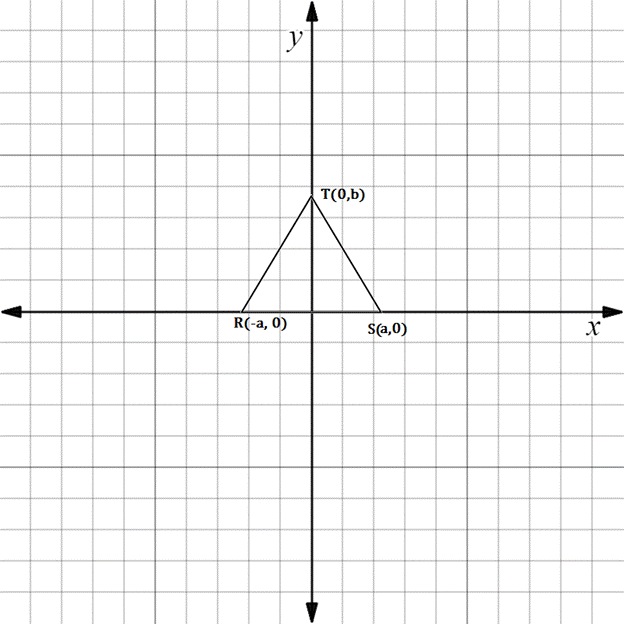Chapter 10.3, Problem 6EElementary Geometry For College St...

7th Edition
Alexander + 2 others
ISBN: 9781337614085

Solutions

Chapter
SectionElementary Geometry For College St...

7th Edition
Alexander + 2 others
ISBN: 9781337614085
Textbook Problem

In Exercises 5 to 10, the real numbers a, b, c, and d are positive.Consider the triangle with vertices at R - a ,   0 , S a ,   0 , and T 0 ,   b . Explain why ∆ R S T is an isosceles triangle.To determine

To explain:

RST is an isosceles triangle.

Explanation

The given diagram is shown below.

To prove RST is an isosceles triangle, we have to prove the following statement.

Isosceles triangle have two equal sides called legs and the third side is called base.

The given vertices are R-a, 0, Sa, 0, and T0, b.

We have to prove RT=TS.

First find the distance between R and T.

x1,y1=R-a, 0

x2,y2=T0,b

Formula for distance between two points

dRT=x2-x12+y2-y12

Substituting the x and y co-ordinates

dRT=0--a2+b-02

On solving this,

dRT=a2+b2

Squaring,

dRT=a2+b2

Now, find the distance between this midpoint T and S

Still sussing out bartleby?

Check out a sample textbook solution.

See a sample solution

The Solution to Your Study Problems

Bartleby provides explanations to thousands of textbook problems written by our experts, many with advanced degrees!

Get Started

Simplify: 1236

Elementary Technical Mathematics

1. Find the first 4 terms of the sequence with nth term

Mathematical Applications for the Management, Life, and Social Sciences

In Exercises 1124, find the indicated limits, if they exist. 19. limx1+x1x(x1)

Applied Calculus for the Managerial, Life, and Social Sciences: A Brief Approach

Simplify the expressions in Exercises 97106. 32/331/6

Finite Mathematics and Applied Calculus (MindTap Course List)

Multiply. (2x21)2

Trigonometry (MindTap Course List)

Differentiate the function. k(r) = er + re

Single Variable Calculus: Early Transcendentals